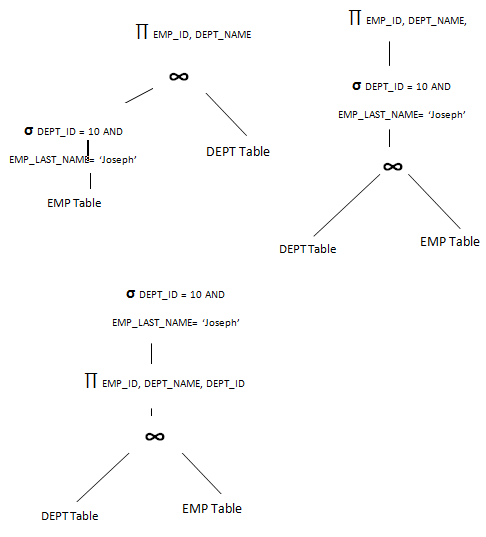# Choice of Evaluation Plans in DBMS

So far we saw how a query is parsed and traversed, how they are evaluated using different methods and what the different costs are when different methods are used. Now the important phase while evaluating a query is deciding which evaluation plan has to be selected so that it can be traversed efficiently. It collects all the statistics, costs, access/ evaluation paths, relational trees etc. It then analyses them and chooses the best evaluation path.

Like we saw in the beginning of this article, same query is written in different forms of relational algebra. Corresponding trees for them too is drawn by DBMS. Statistics for them based on cost based evaluation and heuristic methods are collected. It checks the costs based on the different techniques that we have seen so far. It checks for the operator, joining type, indexes, number of records, selectivity of records, distinct values etc from the data dictionary. Once all these informations are collected, it picks the best evaluation plan.

Have look at below relational algebra and tree for EMP and DEPT.

`∏ EMP_ID, DEPT_NAME (σ DEPT_ID = 10 AND EMP_LAST_NAME = ‘Joseph’ (EMP) ∞DEPT)`

Or

`∏ EMP_ID, DEPT_NAME (σ DEPT_ID = 10 AND EMP_LAST_NAME = ‘Joseph’ (EMP ∞DEPT))`

Or

`σ DEPT_ID = 10 AND EMP_LAST_NAME = ‘Joseph’ (∏ EMP_ID, DEPT_NAME, DEPT_ID (EMP ∞DEPT))`What can be observed here? First tree reduces the number of records for joining and seems to be efficient. But what happens if we have index on DEPT_ID? Then the join between EMP and EMP can also be more efficient. But we see the filter condition on EMP table, we have DEPT_ID = 10, which is index column. Hence first applying selection condition and then join will reduce the number of records as well as make the join more efficient than without index. Next are the projected columns – EMP_ID and DEPT_NAME. they are all distinct values. There cannot be duplicate values for them. But we are selecting those values for DEPT_ID = 10, hence DEPT_NAME has only one value. Hence their selectivity is same as number of employees working for DEPT_ID = 10. But we are selecting only those employees whose last name is ‘Joseph’. Hence the selectivity is min (distinct (employee (DEPT_10)), distinct (employee (DEPT_10, JOSEPH)). Obliviously distinct (employee (DEPT_10, JOSEPH)) would have lesser value. The optimizer decides all these factors for above 3 trees and then decides first tree would be more efficient. Hence it evaluates the query using first tree.

This is how when any query is submitted to DB is traversed and evaluated.

Translate »